StatLect

Classification models

Classification models belong to the class of conditional models, that is, probabilistic models that specify the conditional probability distributions of the output variables given the inputs. The peculiarity of classification models is that in these models the output has a discrete probability distribution (as opposed to regression models, where the output variable is continuous).Types of classification models

There are two different flavors of classification models:

Remember that a Bernoulli random variable can take only two values, either 1 or 0. So, a binary model is used when the output can take only two values.

The Multinoulli distribution is more general. It can be used to model outputs that can take two or more values. If the output variable can takedifferent values, then it is represented as aMultinoulli random vector, that is, a random vector whose realizations have all entries equal to 0, except for the entry corresponding to the realized output value, which is equal to 1Example If the output variable is sex (male of female), then it can be represented as a Bernoulli random variable that takes value 1 for males and 0 for females. It can also be represented as aMultinoulli random vector that takes valuefor males andfor females.

The previous example also shows that a binary classification model (Bernoulli distribution) can always be written as a multinomial model (Multinoulli distribution).

Example If the output variable can belong to one of three classes (red, green or blue), then it can be represented as a Multinoulli random vector whose realizations areMain assumptions and notation

We now introduce the main assumptions, the notation and the terminology we are going to use to present the basics of classification models.

We assume that a sample of dataforis observed by the statistician. The output variables are denoted by, and the associated inputs, which arevectors, are denoted by.

The output can takevalues. In the case of a binary model,,and. In the case of a multinomial model,and, for,is avector whose entries are all equal to zero except for the-th entry, which is equal to.

We assume that there arefunctions, ...,such thatforand. The conditional probability depends not only on the observed output but also on a vector of parameters.

Probabilities need to be non-negative and sum up to 1 (see Probability and its properties). As a consequence, the functionsmust be defined in such a way that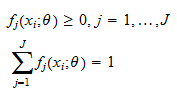for any couple.

Example The logistic classification model is a binary model in which the conditional probability mass function of the outputis a non-linear function of the inputs: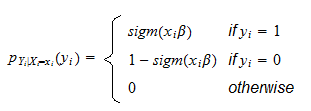whereis avector of coefficients andis the logistic function defined byThus, conditional on, the outputhas a Bernoulli distribution with probability. Using the general notation proposed above and defining, we have: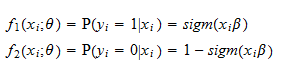It can easily be checked that the probabilities sum up to 1 for anyand any.

Example The multinomial logistic classification model (also called softmax model) is a multinomial model in which the conditional probabilities of the outputs are defined foraswhere to each classcorresponds avector of coefficients. The vector of parametersisThus, conditional on, the outputhas a Multinoulli distribution with probabilitiesEstimation by maximum likelihood

The parameters of a multinomial classification model can be estimated by maximum likelihood. The likelihood of an observationcan be written as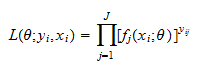whereis the-th component of the Multinoulli vector. Note thattakes value 1 when the output variable belongs to the-th class and 0 otherwise. As a consequence, only one term in the product (the term corresponding to the observed class) can be different from 1. The latter fact is illustrated by the following example.

Example When there are two classes () and the output variable belongs to the second class, we have that the realization of the Multinoulli random vector isThe two components of the vector areand the likelihood is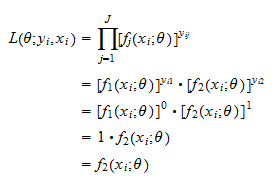Denote thevector of all outputs byand thematrix of all inputs by. If we assume that the observationsin the sample are IID, then the likelihood of the entire sample is equal to the product of the likelihoods of the single observations:and the log-likelihood is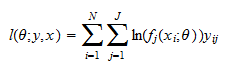The maximum likelihood estimatorof the parametersolvesIn general, there is no analytical solution of this maximization problem and a solution must be found numerically (see the lecture entitled Maximum likelihood algorithm for a detailed explanation of how this can be done). Often, derivatives based algorithms are used (see the aforementioned lecture for an explanation). For several classification models (e.g., the multinomial logistic model introduced in the example above) the use of derivatives based algorithms is facilitated by the fact that the gradient (i.e., the vector of derivatives) of the functionswith respect tocan be computed analytically, which allows to compute analytically also the gradient of the log-likelihood function by using the chain rule:The book

Most of the learning materials found on this website are now available in a traditional textbook format.

Glossary entries
Share